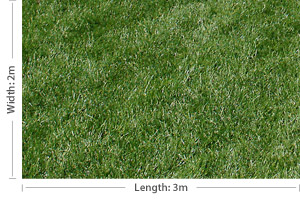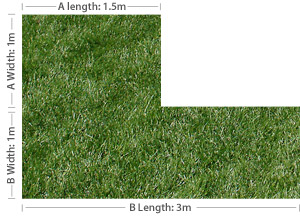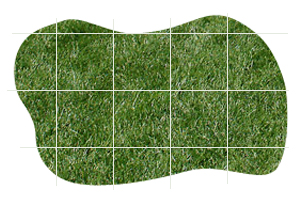# Calculate

Proudly Servicing Newcastle, Maitland, Lake Macquarie, Central Coast, Port Stephens & The Hunter Valley

## Calculate

Finding the area of your yard can be tricky as most yards aren’t perfect shapes. To make the calculation easier we suggest you break your yard down into smaller, easy to calculate sizes.

#### EXAMPLE 1 – Square or Rectangular Yard

To measure a square or rectangular lawn, multiply the width by the length.Area: 3m x 2m = 6m2

#### EXAMPLE 2 – L Shaped Yard

The L shaped yard can be broken into two rectangles. Find the area of each and then add them together. By breaking the yard into smaller shapes finding the area is easy.
Area A: 1.5m x 1m = 1.5m2
Area B: 3m x 1m = 3m2
Total area: A + B = 4.5m2Area A: 1.5m x 1m = 1.5m2
Area B: 3m x 1m = 3m2
Total area: A + B = 4.5m2

#### EXAMPLE 3 – Odd Shaped Yard

If you have a lawn that is an odd shape, the easiest way to measure it is to draw it up with graph paper. Start with the widest and longest points and plot the shape of the garden onto graph paper with each square representing one metre.

Once you have sketched a rough shape onto your graph paper count the number of full squares, estimate the area of the partially filled squares, and add the amounts for an approximate total area. If in doubt, it is better to overestimate the total area than to underestimate.7 full squares = 7m2
11 partially-filled squares = approx. 4m2
Total area = 11m2